GFG App
Open AppBrowser
Continue

# Center of Mass of Different Objects

Center of Mass is an important concept in the area of mechanics. It allows us to deal with very large bodies which will be otherwise very hard to deal with. This concept allows us to consider a very large body as a point mass and perform all the calculations as it is without worrying about its shape and size. For uniform and symmetrical bodies, the Center of mass is usually at the same position as its centroid. But often bodies that are non-uniform and non-symmetrical are encountered. In that case, it is essential to study how to calculate the Center of mass of different bodies of arbitrary shapes and sizes. Let’s study these methods in detail.

### Center of Mass

Consider a body that is made up of many particles. The mass of that body is equal to the total mass of all the particles. In cases where such bodies are undergoing translation, displacement is produced in each and every particle of the body. When such a body is undergoing a motion and a force is acting on it, the force is assumed to be acting on a single point. That single point is termed the Center of mass of the body. It is assumed that the whole mass of the body is concentrated at this point.

### Center of Mass of two Mass Systems

Two mass systems are encountered in a lot of places in real life. A dumbbell can also be considered as a two-mass system. For now, let’s assume that the connection between the two masses that are being considered has zero mass. Let us consider the two masses given in the figure below. The Center of mass of this system is given by,This distance is given from the Center O.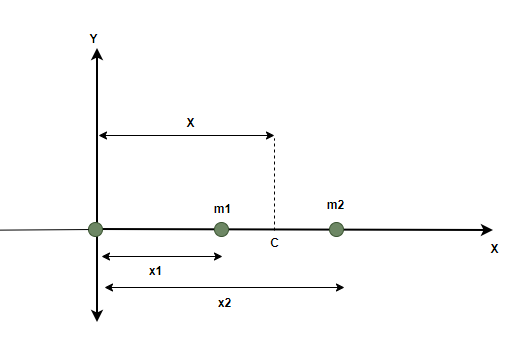The above equation can be seen as a weighted average of the two masses. If both the masses are equal m1 = m2= m.Thus, for particles that are of equal mass, the Center of mass lies at the midpoint. If the system is made up of many particles m1, m2, m3, …..mn along a straight line similar to the previous case. The Center of mass is given by,### General Formula for Center of Mass

Since all systems can be broken down into systems that are made up of many particles at a particular distance from the origin. Up until now, it has been assumed that particles lie on the x-axis. In reality, bodies are three-dimensional. So, the general formula for the center of mass of three-dimensional bodies is given by,### Center of Mass of Different Objects

For objects which are symmetrical and uniform, the Center of mass of such objects lies at their centroid. An example of symmetrical and uniform objects are — rings, circles, squares and cuboids etc. The Center of Mass of some standard shapes is given below.

Equilateral Triangle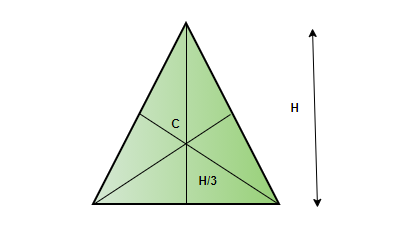ycSemi-Circular Disc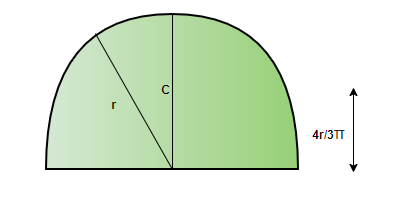ycSemi-Circular Ring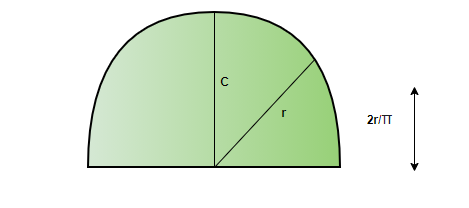ycHemispherical Shell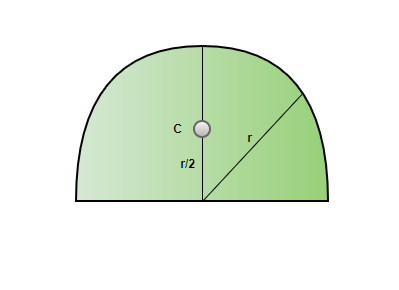ycSolid Hemisphere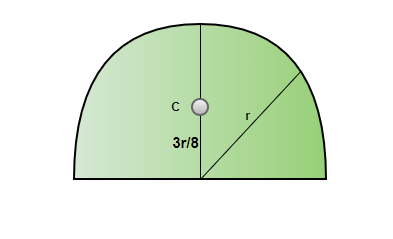yc### Sample Problems

Question 1: Two point masses, m1 = 1Kg and m2 = 2Kg, are located at x = 1 m and x = 2 m respectively. Find the Center of mass.

Solution:

The formula for the Center of mass is given by,

xcmm1 = 1Kg, m2 = 2Kg and x = 1 m and x = 2 m.

M = m1 + m2

⇒ M = 1 + 2 = 3

xcm⇒ xcm⇒ xcm⇒ xcm = 2

Question 2: Twopoint masses, m1 = 4Kg and m2 = 2Kg, are located at vector a = i + j and vector b = -i + j respectively. Find the Center of mass.

Solution:

The formula for the Center of mass in the vector notation is given by,

rcmm1 = 4Kg, m2 = 2Kg and a = i + j, b = -i + j

M = m1 + m2

⇒ M = 4 + 2 = 6

rcm⇒rcm⇒ rcm⇒ rcm⇒ rcmQuestion 3: Twopoint masses, m1 = 4Kg and m2 = 2Kg, are located at vector a = 2i – j and vector b = 3i + 5j respectively. Find the Center of mass.

Solution:

The formula for the Center of mass in the vector notation is given by,

rcmm1 = 4Kg, m2 = 2Kg and a = 2i – j, b = 3i + 5j

M = m1 + m2

⇒ M = 4 + 2 = 6

rcm⇒rcm⇒ rcm⇒ rcm⇒ rcmQuestion 4: A disk of radius R/2 is removed from a bigger disk of mass M of radius R. Find the Center of mass.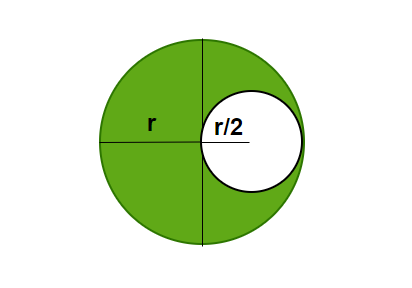Solution:

Since the density of the disk is uniform, the weight is uniformly distributed over all the area.

Mass “m” of the removed disk =The figure shows the center of masses of the remaining portion and the removed portion. Notice that if both of these are taken together, the center of mass should lie at the Center. Let the distance of the center of mass of the remaining portion be “x”.Question 5: Two metal rods of mass “m” and “2 m” are arranged as shown in the figure below. Find the CM of the system if the length of each rod is 1 m.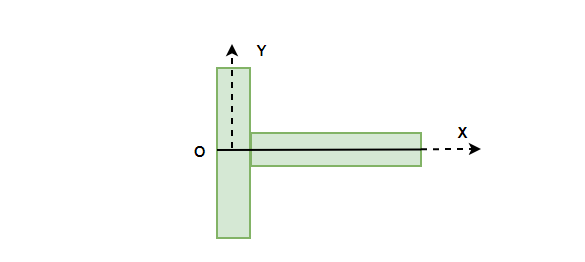Solution:

Since the rods are of uniform density. The Center of mass for each rod lies at its midpoint. Now the whole system can be considered as a system of two point masses kept at their respective Center of masses.

The formula for the Center of mass is given by,

xcmm1 = m, m2 = 2 m and x = 0 m and x = 0.5 m.

M = m1 + m2

⇒ M = 1 m + 2 m = 3 m

xcm⇒ xcm⇒ xcm⇒ xcmMy Personal Notes arrow_drop_up